# matplotlib基础绘图命令之imshow

08/08 19:53

``import matplotlib.pyplot as pltimport numpy as npnp.random.seed(123456789)data = np.random.rand(25).reshape(5, 5)plt.imshow(data)``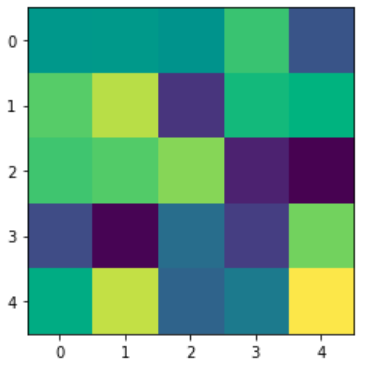imshow方法首先将二维数组的值标准化为0到1之间的值，然后根据指定的渐变色依次赋予每个单元格对应的颜色，就形成了热图。对于热图而言，通常我们还需要画出对应的图例，图例通过colorbar方法来实现，代码如下

``plt.imshow(data)plt.colorbar()``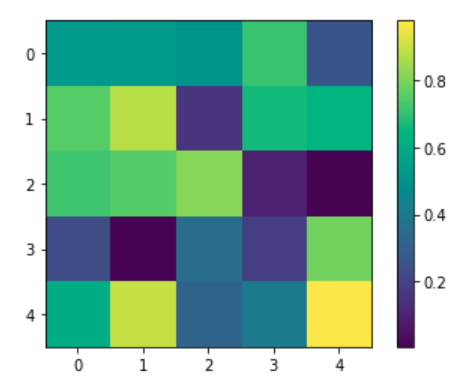imshow方法常用的几个参数如下

1. cmap

cmap是colormap的简称，用于指定渐变色，默认的值为viridis, 在matplotlib中，内置了一系列的渐变色，用法如下

``plt.imshow(data, cmap='Greens')``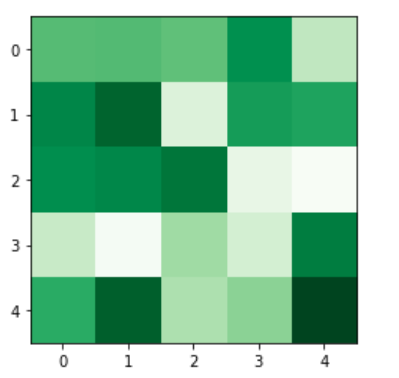https://matplotlib.org/tutorials/colors/colormaps.html

2. aspect

aspect用于指定热图的单元格的大小，默认值为equal,此时单元格用于是一个方块，当设置为auto时，会根据画布的大小动态调整单元格的大小，用法如下

``plt.imshow(data, aspect='auto')``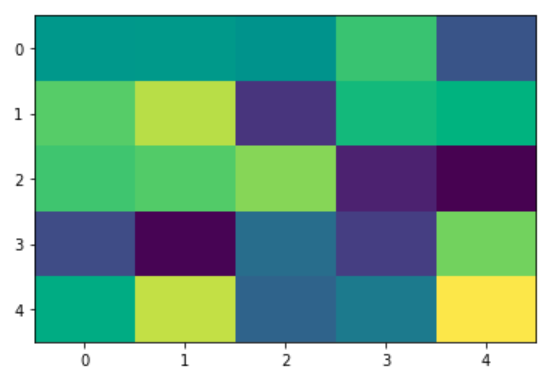3. alpha

alpha参数用于指定透明度，用法如下

``plt.imshow(data, alpha=0.8)``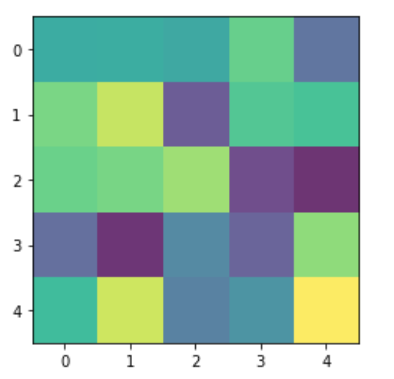4. origin

orign参数指定绘制热图时的方向，默认值为upper,  此时热图的右上角为(0, 0), 当设置为lower时，热图的左下角为(0,0), 用法如下

``plt.imshow(data, origin='lower')``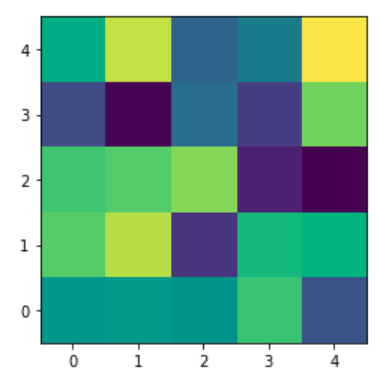5. vmin和vmax

vmin和vmax参数用于限定数值的范围，只将vmin和vmax之间的值进行映射，用法如下

``plt.imshow(data, vmin=-0.8, vmax=0.8)plt.colorbar()``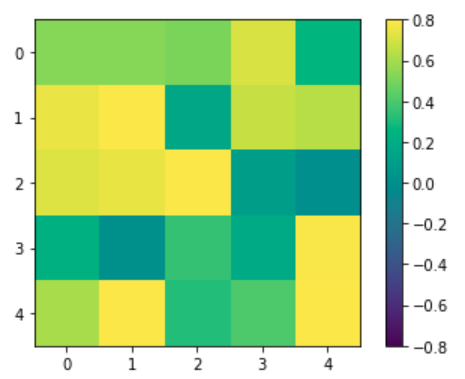6. interpolation

interprolation参数控制热图的显示形式，是一个较难理解的参数，同样的数据，不同取值对应的热图形式如下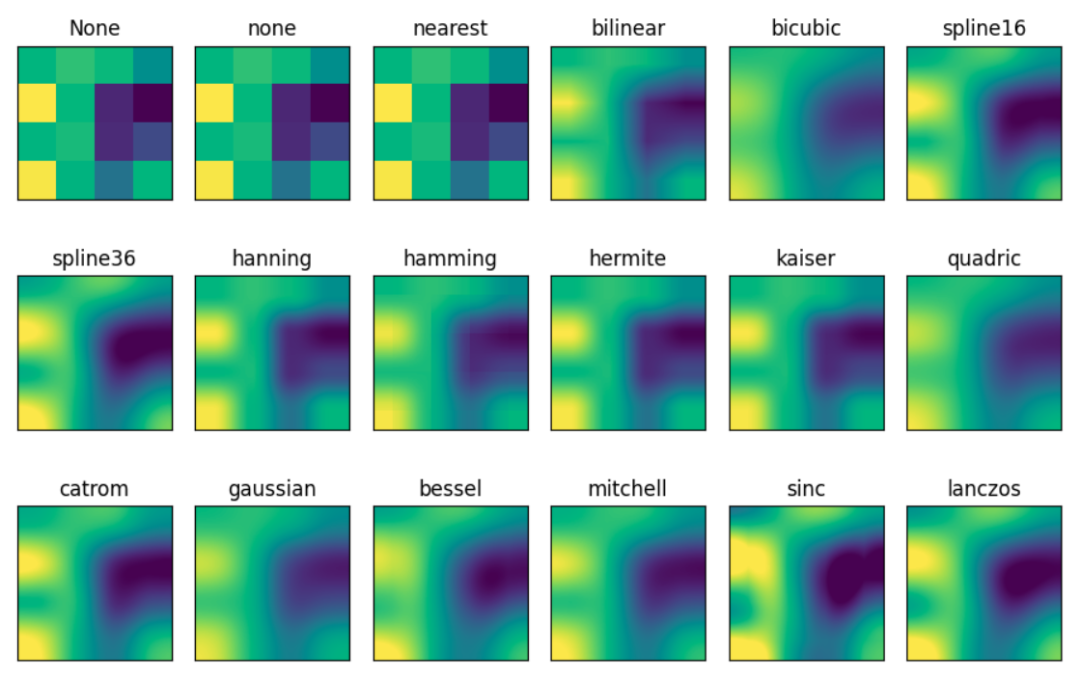7. extent

extent参数指定热图x轴和y轴的极值，取值为一个长度为4的元组或列表，其中，前两个数值对应x轴的最小值和最大值，后两个参数对应y轴的最小值和最大值，用法如下

``plt.imshow(data, extent=(-0.5, 4.5, 4.5, -0.5))``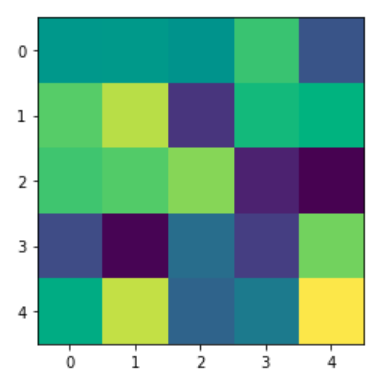``(-0.5, ncol(data) - 0.5, nrow(data)-0.5, 0.5)``

``(-0.5, ncol(data) - 0.5, -0.5, nrow(data)-0.5)``

``plt.imshow(data,extent=(-0.5,5.5,-5.5,0.5))``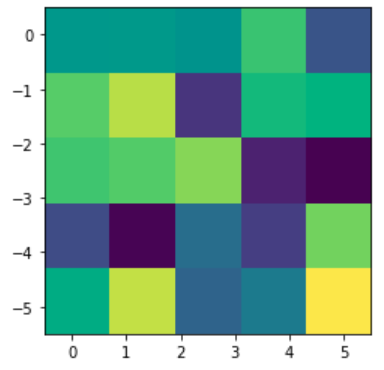``plt.imshow(data)plt.xlim(-1, 5)plt.ylim(5, -1)plt.colorbar()``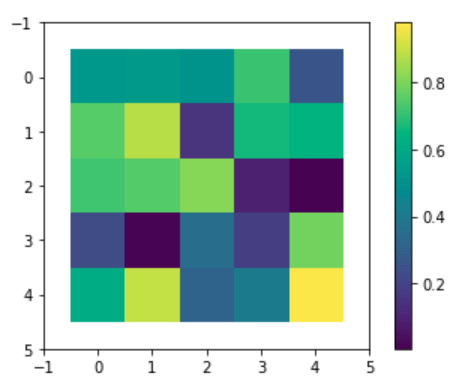·end·

—如果喜欢，快分享给你的朋友们吧—

更多精彩

写在最后0
0 收藏

### 作者的其它热门文章0 评论
0 收藏
0